# Operators in Python - Operation using Symbol

By Vineeth Kumar Published in Python 10-12 mins

# Operators in Python - Operation using SymbolsA character that is used to represent an action in mathematics is an operator. In the same way, python operators are used in performing a particular task on values and variables. There is a specific operation for each symbol or operator.

## What is an operator in python?

Operators are special symbols that substitute the values of the operands. Operators in python act on variables which are called operands. Python is a user-friendly programming language, and if you're interested in learning how to use it effectively, check out the data science course with special python help from top-notch instructors and top-notch resources.

Example:

When you write 'x+y', the operator '+' is used on two operands, 'x' and 'y'.

In this article, you will understand different concepts and types of operators used in python.

## What are the types of operators?

There are seven different types of operators in python. Let us take a look at them individually:

• Arithmetic operator
• Assignment operator
• Comparison or Relational operator
• Logical operator
• Bitwise operator
• Membership operator
• Identity operator

### Arithmetic operator

In python you have seven different arithmetic operators known as binary operators since it is performed on two operands. In simple words, it is a basic mathematical operation.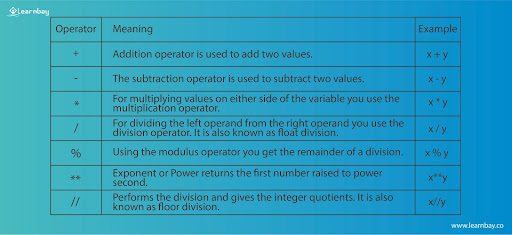Examples of each Arithmetic operator #Addition
x = 10
y = 5
print(x + y)
15

#Subtraction
x = 10
y = 5
print(x - y)
2

#Multiplication
x = 10
y = 5
print(x * y)
50

#Division Float
x = 10
y = 5
print(x / y)
2.0

#Division Floor
x = 10
y = 5
print(x // y)
2.0

#Modules
x = 9
y = 5
print(x % y)
4

#Exponents
x = 7
y = 5
print(x ** y)
16807

### Assignment operator

Assigning values to a variable is called an assignment operator. The result of these operations can later be stored into a variable.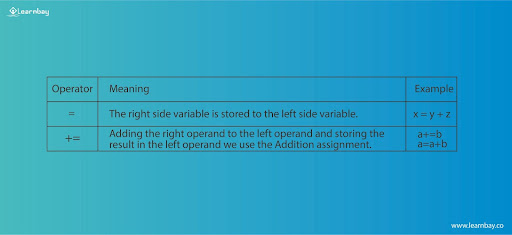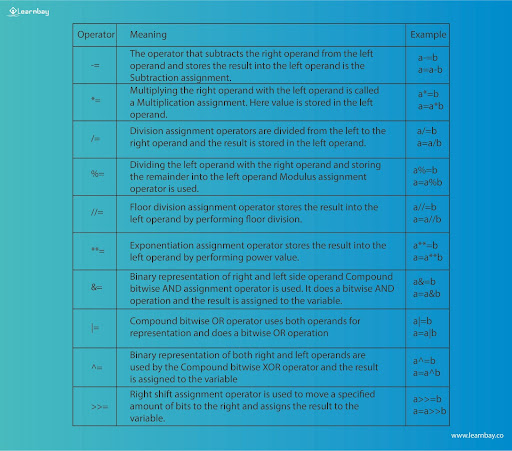Examples for Assignment operators

x = 10
#Assign value
y = x
print(y)

#Add and assign value
y += x
print(y)

#Subtract
y -= x
print(y)

#Multiplication
y *= x
print(y)

#Right shift
y >>= x
print(y)

#left shift
y <<= x
print(y)

10
20
10
100

0.0
0.0
0.0
10.0

### Comparison Operator

Relational operator is also known as the comparison operator where we compare whether two values are the same or which is greater or lesser, etc... Depending on the compared values these operators will result in either True or False.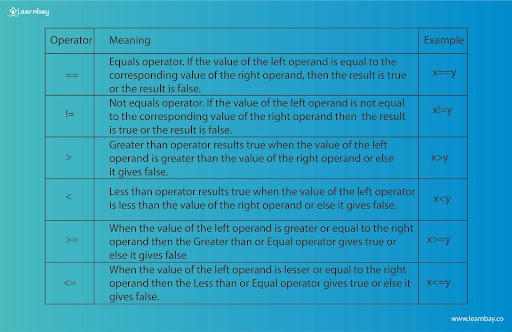### Logical operator

In python symbols or characters used in conditional statements is called Logical operators. It is either True or False. They are capable of performing AND, OR, and NOT operations. You can have a combination of more than a simple compound statement. In Logical Operator, False indicates 0, and True indicates any other number.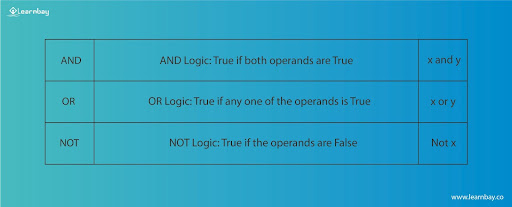• AND Logic: While using this operator, if the first expression is false, further expressions are not evaluated.
• OR Logic: While using this operator, if the first expression is True, further expressions are not evaluated.
• NOT Logic: While using this operator, if the value is True it returns false and vice-versa.

Examples

x = True
y = False

#Print x and y is False
print(x and y)

#Print x or y is True
print(x or y)

#Print not x is False
print(not x)

Output
False
True
False

### Bitwise Operator

Bitwise operators are individual bits (0 and 1) of the operands. These 0 and 1 are referred to as bits or binary digits that represent the values. It is used when integers are transformed into a binary number system. The integers are converted to binary numbers and the operations are executed bit by bit. You can convert decimal numbers to binary numbers and vice-versa.

(Note) : Only Integers work in the Bitwise operator.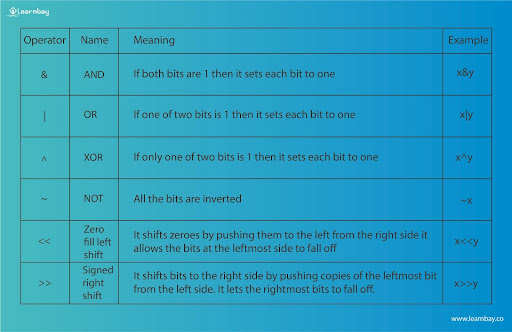Example

| x = 10
y = 4

#Bitwise AND operation
print(x & y)

#Bitwise OR operation
print(x | y)

#Bitwise NOT operation
print(~x)

#Bitwise XOR operation
print(x ^ y)

#Bitwise right shift operation
print(x >> 2)

#Bitwise left shift operation
print(x << 2)

0
14
-11
14
2
40

### Membership Operator

In this we can check whether the elements are present in the sequence or not. It tests membership in strings, lists, tuples, or dictionaries.

There are two membership operators listed below: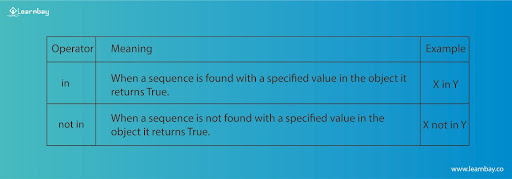Example

x = 32
y = 10
list = [10, 20, 30, 40, 50]

if(x notin list):
print("x is not in the given list")
else:
print("x is in the given list")

if(y in list):
print("y is in the given list")
else:
print("y is not in the given list")

Output
x isnotin the given list
y isin the given list

### Identity Operator

In this we can internally locate the memory location of the object and return an integer number known as the identity number. For checking the memory location of an object we can use the id() function.

There are two types of identity operators: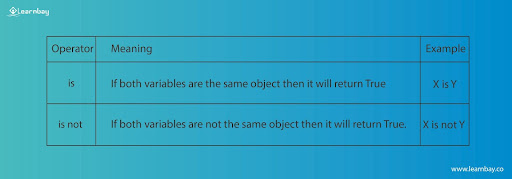Example

| x = 10
y = 20
z = x

print(x isnot y)
print(x is z)

Output
True
True

## Takeaway

Let us summarize, an operator is nothing but a particular task that is performed using special symbols by substituting operands. You've also gone through the various types of operators and examples. It is the basic concept that you work on a day-to-day life while working in python.

Tags

#Python